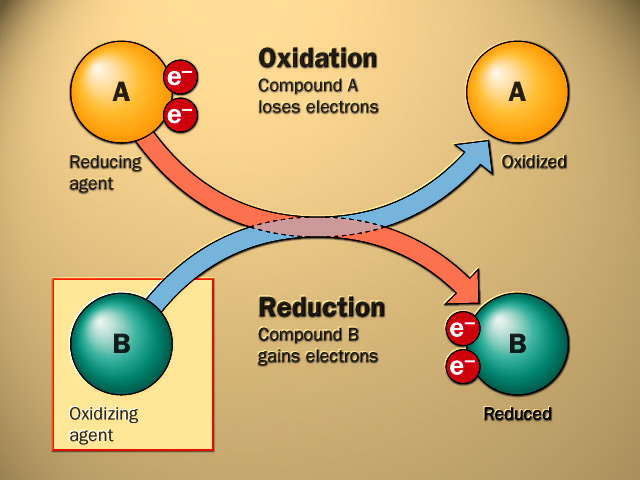Ravela Da Cruz

HS-PS1-2

# Redox Reaction In Basic Solution Study Guide

A redox reaction is also known as a reduction-oxidation reaction. Often, these reactions take place in acidic solutions, although sometimes they can also occur in basic solutions.

Table of content

# Introduction

A redox reaction is commonly known as a reduction-oxidation process. These reactions often take place in acidic solutions and occasionally occur in solutions that are basic. Balancing a redox reaction in a basic solution can easily be done using the half-reaction method. To understand this in detailed form, let's start with its basics.

## WHAT IS A REDOX REACTION?

• An oxidation-reduction reaction, commonly known as a redox reaction, is any chemical process in which the oxidation number of a participating chemical species changes.
• An oxidation-reduction reaction is any chemical reaction in which the oxidation number of a molecule, atom, or ion changes as a result of gaining or losing an electron.
• The creation of hydrogen fluoride is an example of a redox reaction.
• We should break down the reaction to investigate the oxidation and reduction of reactants.Source

## BALANCING REDOX REACTIONS

Balancing of redox reactions happens in two categories. If the solution is acidic, it is treated differently, and if basic, the process of balancing may vary.

## BALANCING REDOX REACTION IN BASIC SOLUTION

Balancing redox reactions in a basic solution is slightly more difficult than the balancing redox reactions in an acidic solution. In the basic solution, we add up H+ and OH- in every half-equation.

## STEPS OF BALANCING

To balance the redox reaction in a basic medium, OH ions and water are added to half of the reactions.

Take the reaction between potassium permanganate and sodium sulfite, for example:

MnO4– + SO32- + H2O → MnO2 +SO42- + OH–

The imbalanced reaction can be divided into two half-reactions, each representing either reduction or oxidation, just as it can in acidic media.

Reduction: 3 e− + 2 H2O + MnO4− → MnO2 + 4 OH−

Oxidation: 2 OH− + SO32− → SO42− + H2O + 2 e−

When the number of electrons in the two half-reactions are balanced, the result is:

6 e− + 4 H2O + 2 MnO4− → 2 MnO2 + 8 OH−

6 OH− + 3 SO32− → 3 SO42− + 3 H2O + 6 e−

The balanced equation is obtained by adding these two half-reactions together:

2 MnO4– + 3 SO32- + H2O → 2 MnO2 + 3 SO42- + 2 OH–

# SUMMARY

• The loss of electrons due to the transfer of electrons in the oxidation state is called oxidation. In contrast, the gain of electrons in the oxidation state is said to be the reduction process.
• The electron acceptor always undergoes a reduction process called an oxidizing agent. The electron donor undergoes an oxidation process in a redox reaction called a reducing agent.
• We add up OH- ions to the equation when the redox reaction is in a basic solution.
• The half reaction method is more versatile if no acid or bases are present.

## FAQs

1. How do you balance a redox reaction by the half-reaction method?

1. First, split up the equation into two halves.
2. Balance each half-equation for its charge and mass.
3. Evaluate the number of electrons and then combine both equations.

2. How do you balance redox reactions by the ion-electron method in a basic medium?

• Divide the reaction into two halves to balance in basic solution.
• Balance each reaction with O- rather than H+ in H2O
• Balance the hydrogen atom with H+
• Add OH- ions on both sides to neutralize the equation.
• Combine OH- and H+ to create H2O
• Simplify the equation
• Combine both half equations.

3. How do you balance half equations?

Balancing half equations can be done by balancing the atoms separately. The number of electrons lost is equalized to the number of electrons gained.

4. What is step 1 in the ion-electron method for balancing redox reactions?

Writing down the unbalanced equations and then separating the reactions into two halves as per their undergoing process.

We hope you enjoyed studying this lesson and learned something cool about Redox Reaction In Basic Solutions! Join our Discord community to get any questions you may have answered and to engage with other students just like you! Don't forget to download our app to experience our fun VR classrooms - we promise it makes studying much more fun!😎

1. Balance Redox Reaction in basic Solution: https://socratic.org/questions/how-do-you-balance-redox-reactions-in-basic-solution. Accessed 3rd March 2022.
2. How to Balance Redox Reactions: https://blog.cambridgecoaching.com/how-to-balance-redox-reactions-in-acidic-and-basic-solutions. Accessed 3rd March 2022.
3. Balancing Redox Reactions: https://flexbooks.ck12.org/cbook/ck-12-chemistry-flexbook-2.0/section/22.11/primary/lesson/half-reaction-method-in-basic-solution-chem/. Accessed 3rd March 2022.
4. Balancing Redox Equations: https://courses.lumenlearning.com/introchem/chapter/balancing-redox-equations-2/. Accessed 3rd March 2022.
5. Balancing Redox Reactions: https://www.ck12.org/c/chemistry/balancing-redox-reactions%3A-half-reaction-method-in-basic-solution/lesson/Half-Reaction-Method-in-Basic-Solution-CHEM/.Accessed 3rd March 2022.